Maths-
General
Easy

Question

# 50 animals are there in a shed. Some of the animals have 2 legs and the rest of them have 4 legs. In total there are 172 legs. Frame the system of linear equations and use elimination method to solve .Hint:

## The correct answer is: 24

### Ans :- The no. of 2 leg animals are 26 and no. of 4 leg animals are 24.Explanation :-Step 1:- Frame the equationslet the no. of 2 leg animals be x and let the number of 4 leg animals be yGiven total no. of animals = no. of 2 leg animals + no. of 4 leg animals = 50i. e x + y = 50 — eq1Given no. of legs = 2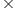no. of 2 leg animals  + 4no. of 4 leg animals = 172.i. e 2x+4y = 172 — eq2So the equations are x + y = 50 and 2x + 4y = 172Step 2 :- eliminate x to find value of yDoing  eq2 - 2eq1 to eliminate xWe get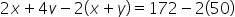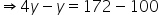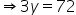∴ y = 24 Step 3:- substitute the value of y in eq1 to get the value of x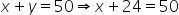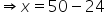∴ x = 26 ∴The no. of 2 leg animals are 26 and no. of 4 leg animals are 24.#### With Turito Foundation.#### Get an Expert Advice From Turito.